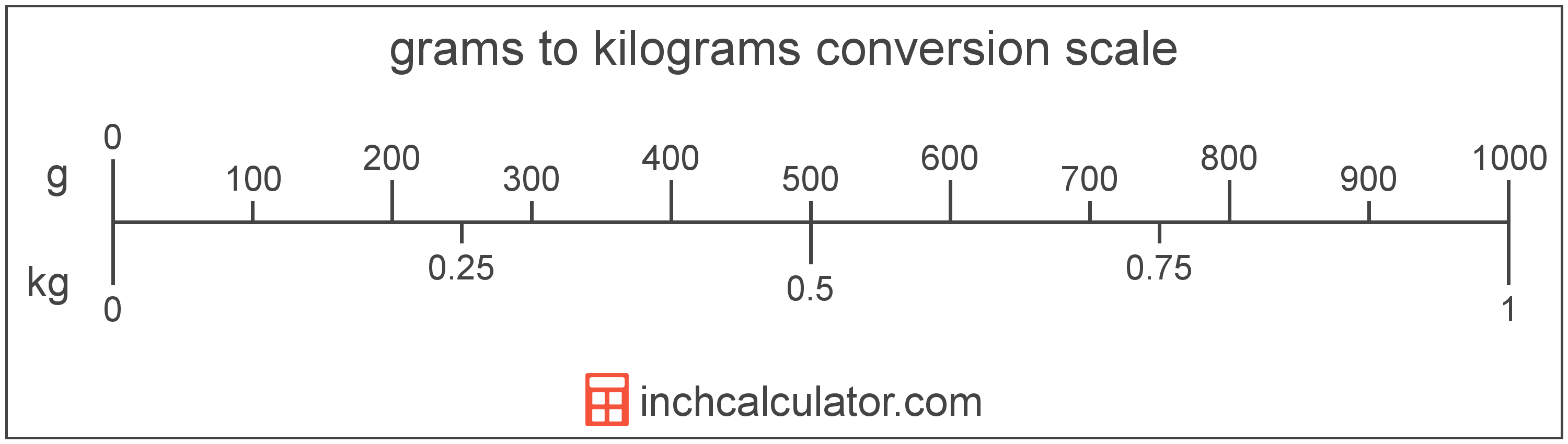# Grams to Kilograms Converter

Enter the weight in grams below to get the value converted to kilograms.

Results in Kilograms:1 g = 0.001 kg

Do you want to convert kilograms to grams?

## How to Convert Grams to Kilograms

To convert a measurement in grams to a measurement in kilograms, divide the weight by the following conversion ratio: 1,000 grams/kilogram.

Since one kilogram is equal to 1,000 grams, you can use this simple formula to convert:

kilograms = grams ÷ 1,000

The weight in kilograms is equal to the weight in grams divided by 1,000.

For example, here's how to convert 5,000 grams to kilograms using the formula above.
kilograms = (5,000 g ÷ 1,000) = 5 kg## What Is a Gram?

A gram is a unit of mass equal to 1/1,000 of a kilogram or 0.035274 ounces, and is equivalent to the mass of one cubic centimeter, or one milliliter, of water.

The gram, or gramme, is an SI unit of weight in the metric system. Grams can be abbreviated as g; for example, 1 gram can be written as 1 g.

## What Is a Kilogram?

One kilogram is equal to 1,000 grams, 2.204623 pounds, or 1/1,000 of a metric ton.

The formal definition of the kilogram changed in 2019. One kilogram was previously equal to the mass of the platinum-iridium bar, known as the International Prototype of the Kilogram, which was stored in Sèvres, France.

The 2019 SI brochure now defines the kilogram using the Planck constant, and it is defined using the meter and second. It is equal to the mass of 1,000 cubic centimeters, or milliliters, of water.

The kilogram, or kilogramme, is the SI base unit for weight and is also a multiple of the gram. In the metric system, "kilo" is the prefix for thousands, or 103. Kilograms can be abbreviated as kg; for example, 1 kilogram can be written as 1 kg.

## Gram to Kilogram Conversion Table

Table showing various gram measurements converted to kilograms.
Grams Kilograms
1 g 0.001 kg
2 g 0.002 kg
3 g 0.003 kg
4 g 0.004 kg
5 g 0.005 kg
6 g 0.006 kg
7 g 0.007 kg
8 g 0.008 kg
9 g 0.009 kg
10 g 0.01 kg
20 g 0.02 kg
30 g 0.03 kg
40 g 0.04 kg
50 g 0.05 kg
60 g 0.06 kg
70 g 0.07 kg
80 g 0.08 kg
90 g 0.09 kg
100 g 0.1 kg
200 g 0.2 kg
300 g 0.3 kg
400 g 0.4 kg
500 g 0.5 kg
600 g 0.6 kg
700 g 0.7 kg
800 g 0.8 kg
900 g 0.9 kg
1,000 g 1 kg

## References

1. International Bureau of Weights and Measures, The International System of Units, 9th Edition, 2019, https://www.bipm.org/documents/20126/41483022/SI-Brochure-9-EN.pdf